## Tuesday, May 31, 2016

### More on `plot` method for object class `"fitMk"`

I've just been playing with the S3 `plot` method that I added to phytools. Here's how it can be used for characters with two, three, four, five, or more states. I'm going to use simulated data just for illustrative purposes, except for one Anolis example at the very end:

``````library(phytools)
## two states
tree<-pbtree(n=26,tip.label=letters,scale=1)
Q<-matrix(c(-2,2,1,-1),2,2)
rownames(Q)<-colnames(Q)<-c("aquatic","terrestrial")
x<-as.factor(sim.history(tree,Q)\$states)
``````
``````## Note - the rate of substitution from i->j should be given by Q[j,i].
## Done simulation(s).
``````
``````fit2<-fitMk(tree,x,model="ARD")
plot(fit)
``````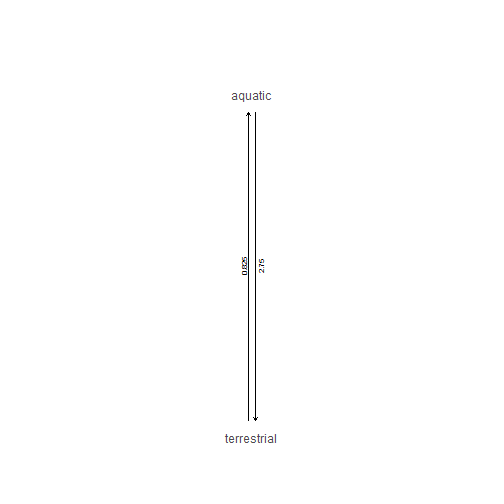``````## three states
tree<-pbtree(n=100,scale=1)
Q<-matrix(c(-1,1,0,0,-1,1,0,1e-12,-1e-12),3,3)
rownames(Q)<-colnames(Q)<-paste("state",c("I","II","III"))
x<-as.factor(sim.history(tree,Q,anc="state I")\$states)
``````
``````## Note - the rate of substitution from i->j should be given by Q[j,i].
## Done simulation(s).
``````
``````fit3<-fitMk(tree,x,model="ARD")
fit3
``````
``````## Object of class "fitMk".
##
## Fitted (or set) value of Q:
##             state I  state II state III
## state I   -0.631994  0.382593  0.249401
## state II   0.000000 -0.731563  0.731563
## state III  0.000000  0.000000  0.000000
##
## Fitted (or set) value of pi:
##   state I  state II state III
## 0.3333333 0.3333333 0.3333333
##
## Log-likelihood: -38.374895
``````
``````plot(fit3,cex.traits=0.8,cex.rates=0.8,mar=rep(0,4))
``````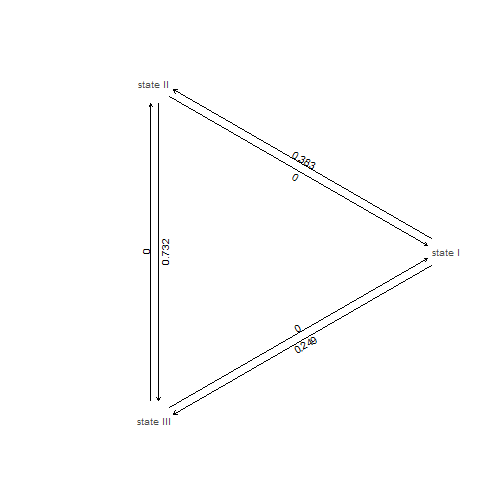``````plot(fit3,cex.traits=0.8,cex.rates=0.8,mar=rep(0,4),
show.zeros=FALSE)
``````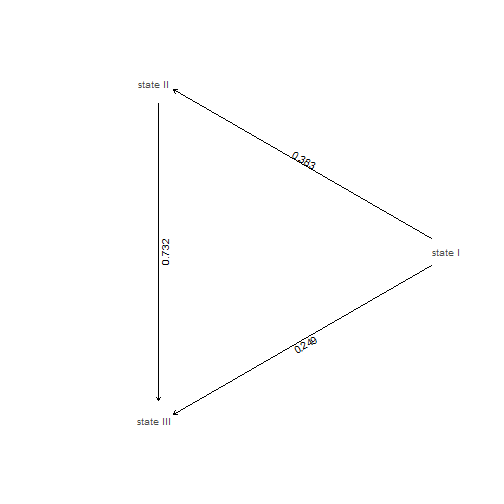``````## four states
tree<-pbtree(n=200,scale=1)
Q<-matrix(c(-1,1,0,0,
0,-1,1,0,
0,0,-1,1,
1,0,0,-1),4,4)
rownames(Q)<-colnames(Q)<-letters[1:4]
x<-as.factor(sim.history(tree,Q)\$states)
``````
``````## Note - the rate of substitution from i->j should be given by Q[j,i].
## Done simulation(s).
``````
``````fit4<-fitMk(tree,x,model="ARD")
plot(fit4,show.zeros=FALSE)
``````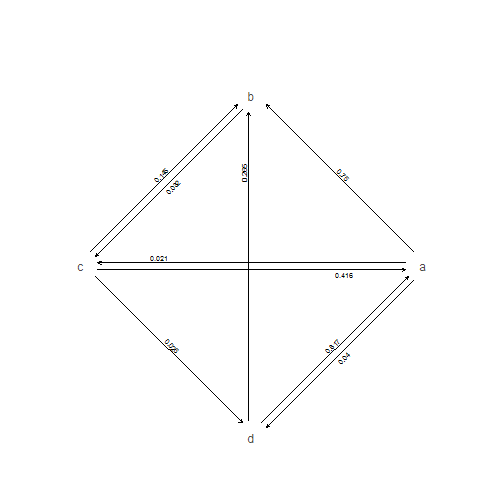``````## five states
Q<-matrix(c(0,3,2,1,0,
3,0,3,2,1,
2,3,0,3,2,
1,2,3,0,3,
0,1,2,3,0),5,5)
diag(Q)<--colSums(Q)
rownames(Q)<-colnames(Q)<-levels<-
c("some","slightly","longer","named","states")
x<-factor(sim.history(tree,Q)\$states,levels=levels)
``````
``````## Done simulation(s).
``````
``````fit5<-fitMk(tree,x,model="SYM")
plot(fit5,cex.traits=0.8)
``````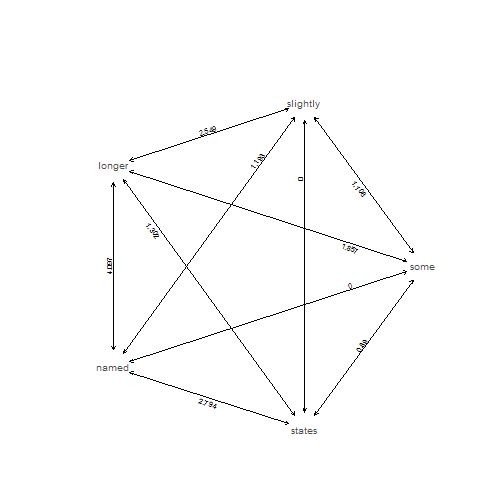``````## ten states
Q<-matrix(rep(1,100),10,10)
diag(Q)<-0
diag(Q)<--colSums(Q)
rownames(Q)<-colnames(Q)<-letters[1:10]
x<-sim.history(tree,Q)\$states
``````
``````## Done simulation(s).
``````
``````fitER<-fitMk(tree,x,model="ER")
plot(fitER)
``````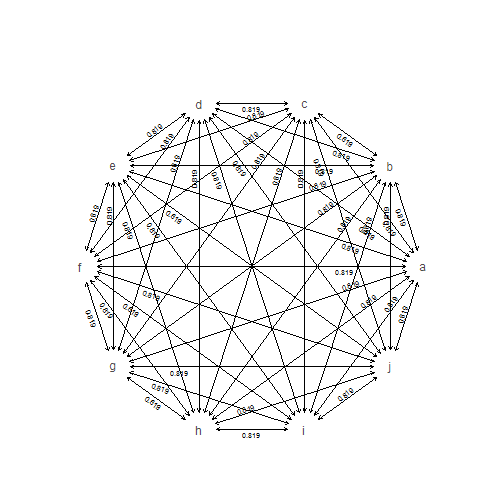Finally, let's load the Anolis data:

``````data(anoletree)
anoletree
``````
``````##
## Phylogenetic tree with 82 tips and 81 internal nodes.
##
## Tip labels:
##  Anolis_ahli, Anolis_allogus, Anolis_rubribarbus, Anolis_imias, Anolis_sagrei, Anolis_bremeri, ...
##
## The tree includes a mapped, 6-state discrete character with states:
##  CG, GB, TC, TG, Tr, Tw
##
## Rooted; includes branch lengths.
``````
``````x<-getStates(anoletree,"tips")
fitARD<-fitMk(anoletree,x,model="ARD")
``````
``````## Warning in log(comp[1:M + N]): NaNs produced

## Warning in log(comp[1:M + N]): NaNs produced

## Warning in log(comp[1:M + N]): NaNs produced

## Warning in log(comp[1:M + N]): NaNs produced

## Warning in log(comp[1:M + N]): NaNs produced

## Warning in log(comp[1:M + N]): NaNs produced

## Warning in log(comp[1:M + N]): NaNs produced

## Warning in log(comp[1:M + N]): NaNs produced

## Warning in log(comp[1:M + N]): NaNs produced

## Warning in log(comp[1:M + N]): NaNs produced

## Warning in log(comp[1:M + N]): NaNs produced

## Warning in log(comp[1:M + N]): NaNs produced

## Warning in log(comp[1:M + N]): NaNs produced

## Warning in log(comp[1:M + N]): NaNs produced

## Warning in log(comp[1:M + N]): NaNs produced

## Warning in log(comp[1:M + N]): NaNs produced

## Warning in log(comp[1:M + N]): NaNs produced

## Warning in log(comp[1:M + N]): NaNs produced

## Warning in log(comp[1:M + N]): NaNs produced

## Warning in log(comp[1:M + N]): NaNs produced

## Warning in log(comp[1:M + N]): NaNs produced

## Warning in log(comp[1:M + N]): NaNs produced

## Warning in log(comp[1:M + N]): NaNs produced

## Warning in log(comp[1:M + N]): NaNs produced

## Warning in log(comp[1:M + N]): NaNs produced

## Warning in log(comp[1:M + N]): NaNs produced
``````
``````plot(fitARD,show.zeros=FALSE,tol=1e-3,signif=4)
``````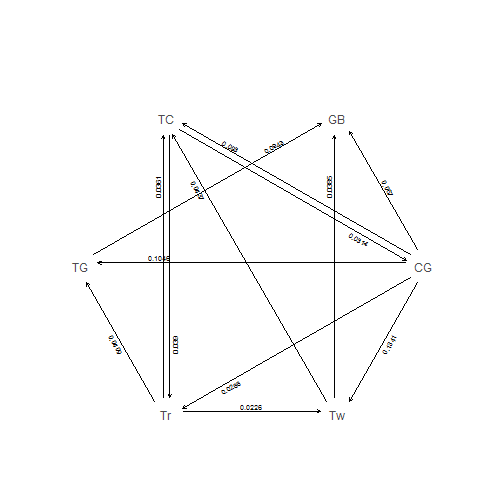(Note that I wouldn't put too much credence in this particular result. This is just way too many parameters to estimate from a tree with 82 tips!)

That's it.

### Plotting method for fitted discrete character (Mk) models

Today I've been working on an S3 `plot` method for objects of class `"fitMk"`. This is similar to what I showed before (1, 2) for objects of class `"fitPagel"`, except that this time it is for a single discrete character, but potentially with multiple states (theoretically an arbitrary number).

Here is what I have so far:

``````plot.fitMk<-function(x,...){
if(hasArg(main)) main<-list(...)\$main
else main<-NULL
if(hasArg(cex.main)) cex.main<-list(...)\$cex.main
else cex.main<-1.2
if(hasArg(cex.traits)) cex.traits<-list(...)\$cex.traits
else cex.traits<-1
if(hasArg(cex.rates)) cex.rates<-list(...)\$cex.rates
else cex.rates<-0.6
if(hasArg(show.zeros)) show.zeros<-list(...)\$show.zeros
else show.zeros<-TRUE
if(hasArg(tol)) tol<-list(...)\$tol
else tol<-1e-6
Q<-matrix(NA,length(x\$states),length(x\$states))
Q[]<-c(0,x\$rates)[x\$index.matrix+1]
diag(Q)<-0
spacer<-0.1
plot.new()
par(mar=c(1.1,1.1,3.1,1.1))
xylim<-c(-1.2,1.2)
plot.window(xlim=xylim,ylim=xylim,asp=1)
if(!is.null(main)) title(main=main,cex.main=cex.main)
nstates<-length(x\$states)
step<-360/nstates
angles<-seq(0,360-step,by=step)/180*pi
v.x<-cos(angles)
v.y<-sin(angles)
for(i in 1:nstates) for(j in 1:nstates)
if(if(!isSymmetric(Q)) i!=j else i>j){
dx<-v.x[j]-v.x[i]
dy<-v.y[j]-v.y[i]
slope<-abs(dy/dx)
shift.x<-0.02*sin(atan(dy/dx))*sign(j-i)*if(dy/dx>0) 1 else -1
shift.y<-0.02*cos(atan(dy/dx))*sign(j-i)*if(dy/dx>0) -1 else 1
s<-c(v.x[i]+spacer*cos(atan(slope))*sign(dx)+
if(isSymmetric(Q)) 0 else shift.x,
v.y[i]+spacer*sin(atan(slope))*sign(dy)+
if(isSymmetric(Q)) 0 else shift.y)
e<-c(v.x[j]+spacer*cos(atan(slope))*sign(-dx)+
if(isSymmetric(Q)) 0 else shift.x,
v.y[j]+spacer*sin(atan(slope))*sign(-dy)+
if(isSymmetric(Q)) 0 else shift.y)
if(show.zeros||Q[i,j]>tol){
if(abs(diff(c(i,j)))==1||abs(diff(c(i,j)))==(nstates-1))
text(mean(c(s,e))+1.5*shift.x,
mean(c(s,e))+1.5*shift.y,
round(Q[i,j],3),cex=cex.rates,
srt=atan(dy/dx)*180/pi)
else
text(mean(c(s,e))+0.3*diff(c(s,e))+
1.5*shift.x,
mean(c(s,e))+0.3*diff(c(s,e))+
1.5*shift.y,
round(Q[i,j],3),cex=cex.rates,
srt=atan(dy/dx)*180/pi)
arrows(s,s,e,e,length=0.05,
code=if(isSymmetric(Q)) 3 else 2)
}
}
text(v.x,v.y,x\$states,cex=cex.traits,
col=make.transparent("black",0.7))
}
``````

Now, let's try it!

Here I have a 300 taxon tree & a 6-state character:

``````library(phytools)
tree
``````
``````##
## Phylogenetic tree with 300 tips and 299 internal nodes.
##
## Tip labels:
##  t62, t133, t134, t38, t267, t268, ...
##
## Rooted; includes branch lengths.
``````
``````y
``````
``````##  t62 t133 t134  t38 t267 t268 t122 t154 t168 t169 t127 t190 t191 t226 t227
##    F    F    F    F    F    F    F    F    F    F    F    F    F    F    F
##  t84  t78  t51 t299 t300 t143  t70  t64 t273 t274 t255 t111 t177 t178  t63
##    F    F    F    F    F    F    F    F    F    F    F    F    F    F    F
##  t23 t175 t176  t71  t49 t265 t266 t103 t104  t97  t98  t35  t36 t114 t115
##    F    F    E    F    E    F    E    F    E    F    F    F    F    E    E
##  t31  t60 t172 t187 t188  t48 t201 t202 t277 t278 t230 t159 t160  t24 t109
##    E    E    E    E    E    F    D    D    D    D    D    D    D    D    D
## t110  t82  t16  t89  t90  t26  t27 t141 t142  t91  t92  t47 t128 t129 t196
##    C    D    D    E    E    E    D    D    D    D    E    D    D    D    D
## t247 t248  t39  t44  t45  t17 t222 t223   t5   t6  t30  t76  t77  t72  t73
##    D    D    C    E    E    D    D    D    D    D    D    D    D    C    C
##  t74  t75 t297 t298 t117 t118 t259 t260 t189 t245 t246  t53  t54  t55  t56
##    B    B    C    C    C    C    C    C    C    C    C    C    C    C    C
##  t11 t231 t232 t214 t184 t279 t280 t112 t113 t289 t290  t15 t217 t218  t14
##    B    C    C    C    C    B    B    B    B    D    D    D    C    C    D
## t144 t145  t20  t13  t33 t228 t229 t221 t236 t237 t135  t99  t66  t67 t287
##    D    D    C    F    F    E    E    E    F    F    F    F    F    F    F
## t288 t130 t249 t250  t37 t194 t195  t58 t185 t186 t173 t174  t46  t83 t234
##    F    E    F    F    F    F    F    E    E    E    F    F    F    F    F
## t235 t233 t257 t258 t252 t132 t164 t165 t161   t1   t3  t32  t52 t207 t208
##    F    F    F    F    F    F    F    F    F    D    C    C    B    B    B
##  t22 t215 t216 t256 t293 t294 t116 t105 t106 t179 t291 t292  t57  t93  t94
##    B    A    A    B    B    B    B    C    C    D    D    D    E    F    F
##  t12  t79  t80 t285 t286  t95  t96  t50  t18  t19  t21 t263 t264 t209 t210
##    D    C    C    C    C    C    C    C    D    D    C    C    C    D    D
## t182 t183  t28 t149 t150  t42  t43 t136 t156 t261 t262 t244 t131 t137 t253
##    D    D    D    D    D    E    E    D    D    D    D    D    D    D    C
## t254 t251 t281 t282 t203 t204  t25 t275 t276  t88   t4 t170 t283 t284 t146
##    C    C    C    C    C    D    D    D    D    D    D    D    E    E    E
##  t81 t269 t270 t211   t8 t238 t239 t171  t61  t85  t86   t7 t242 t243 t180
##    E    D    D    D    C    C    C    C    C    C    C    B    C    C    C
## t181 t123 t124  t65 t147 t148 t102 t138 t139 t295 t296 t197 t198 t192 t193
##    C    B    B    B    B    B    B    C    C    D    D    B    B    B    B
## t120 t121 t219 t220  t34 t166 t167 t224 t225 t151 t152 t119  t41  t68  t69
##    C    C    E    E    D    C    C    D    D    C    C    C    C    C    B
##   t2   t9  t10 t240 t241 t271 t272 t157 t158  t59 t153 t199 t200 t162 t163
##    B    C    C    C    C    C    C    D    D    C    C    C    C    C    C
##  t87  t40 t125 t126 t107 t108  t29 t155 t212 t213 t140 t205 t206 t100 t101
##    D    D    C    C    C    C    C    C    C    C    D    C    C    C    C
## Levels: A B C D E F
``````

Now let's fit a symmetric (`model="SYM"`) and all-rates-different (`model="ARD"`) models and then visualize the results:

``````fitSYM<-fitMk(tree,y,model="SYM")
plot(fitSYM,main="Fitted \"SYM\" model")
``````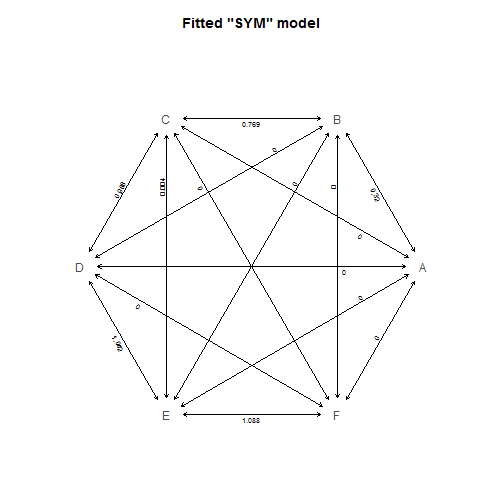``````fitARD<-fitMk(tree,y,model="ARD")
plot(fitARD,main="Fitted \"ARD\" model")
``````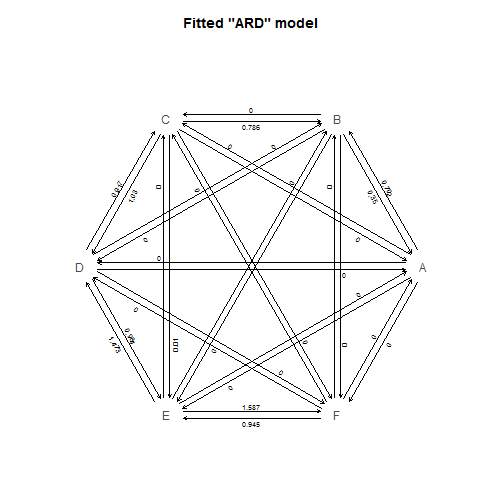I have not yet included the feature to set arrow widths by estimated rates; however this preliminary version does permit us to avoid plotting rates that are not different from zero, for instance:

``````plot(fitSYM,show.zeros=FALSE,main="Fitted \"SYM\" model (no zeros)")
``````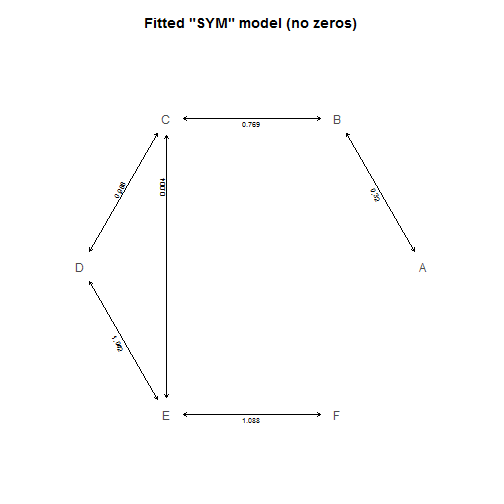``````plot(fitARD,show.zeros=FALSE,main="Fitted \"ARD\" model (no zeros)")
``````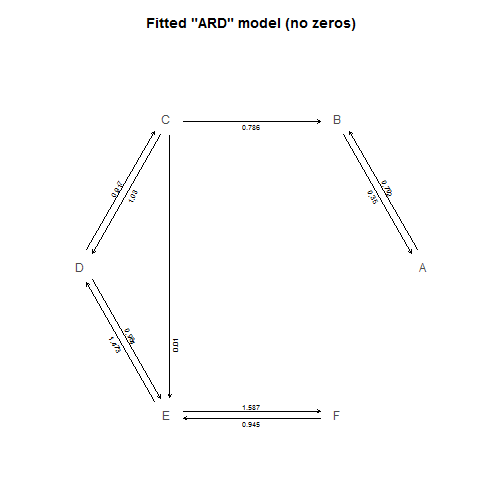Note that, at least in this case, the fitted models are quite similar to the generating model, which is a constant-rate, ordered, reversible model: `A <-> B <-> C <-> D <-> E <-> F <-> G`.

This is just for objects of class `"fitMk"` now; however it could easily be modified to work with `ace` or `fitDiscrete` in the geiger package.

The data were simulated as follows:

``````tree<-pbtree(n=300,scale=1)
Q<-cbind(c(-1,1,0,0,0,0),
c(1,-2,1,0,0,0),
c(0,1,-2,1,0,0),
c(0,0,1,-2,1,0),
c(0,0,0,1,-2,1),
c(0,0,0,0,1,-1))
rownames(Q)<-colnames(Q)<-LETTERS[1:nrow(Q)]
y<-as.factor(sim.history(tree,Q)\$states)
``````